SCS (NRCS) Hydrographs

This article will explain exactly how the NRSC hydrographs are constructed and hopefully will give you a better understanding of what goes on behind the scenes of the software.

Hydrology Studio uses the Unit Hydrograph Method for calculating NRCS runoff hydrographs. This method is the same approach as used in the original TR-20. There are basically four steps involved:

1. A Design Storm is selected (Typically an NRCS 24-hr distribution)
2. A Unit Hydrograph is constructed based on the drainage area’s characteristics, i.e., Curve Number (CN), Tc, etc.
3. The Design Storm is applied to the Unit Hydrograph by multiplying each ordinate of the Design Storm (after it has been converted to an excess hyetograph) by each ordinate of the Unit Hydrograph, creating a series of Individual Hydrographs. A lot of them. For example, using a 2-minute Time Interval produces 720 individual Unit Hydrographs.
4. The Individual Hydrographs are summed to create the Final Runoff Hydrograph.The Final Runoff Hydrograph is the summation of the Individual Hydrographs

NRCS Unit Hydrograph

A unit hydrograph is a hydrograph resulting from 1 inch of rainfall excess on a watershed over a given time interval. It is not the final runoff hydrograph but reflects the watershed characteristics. Once a unit hydrograph of a particular watershed is known, any design storm can be applied to it for computing the final runoff hydrograph.

The peak discharge for the unit graph is computed as: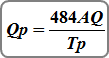Where:
Qp = peak outflow (cfs)
484 = Shape Factor (0.208 metric)
A = area (sq. miles)
Q = total excess precipitation, 1 inch (1 mm)
Tp = time to peak (hrs)

The shape factor is a user-definable variable. The default value is set to 484 and creates a unit hydrograph that has 3/8 of its area under its rising limb. This factor is higher in mountainous watersheds, for example, 600, while in flat, sandy areas, will be lower, around 300. The Delmarva peninsula in Delaware uses 284.

The Time to Peak, Tp, and the Time Base, Tb, are what determines the characteristics of the unit hydrograph. These values are computed as follows: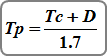Where:
Tp = time to peak (hrs)
Tc = time of concentration (hrs)
D = time interval (hrs)
Tc = 1.67 x Lag Time (L)Where:
L = lag time (hrs)
l = hydraulic length (ft)
S = (1000 / CN) – 10
Y = basin slope (%)
CN = SCS curve number

Time Base = 2.67Tp

Where:
Tb = time base (hrs)
Tp = time to peak (hrs)

It should be noted that the program will adjust the Time to Peak so that it coincides with the current Time Interval.

Design Storms

Hydrology Studio offers several built-in design storms including those which you can customize. Most of which are the NRCS 24-hr and 6-hr standard distributions. But other options include the IDF-based Synthetic Storms, Huff and as many as ten custom storms that you input directly. See Custom Design Storms.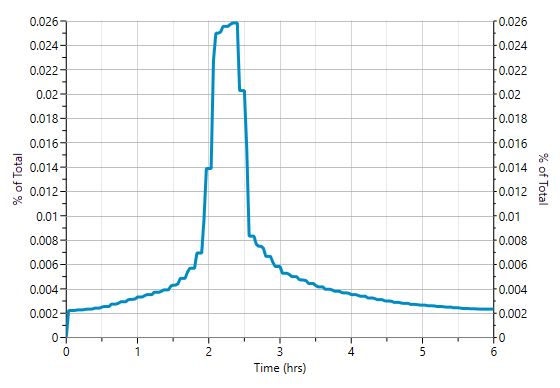This is the NRCS Standard 6-hour dimensionless design storm distribution.

NRCS 24-Hour Distributions

This hydrology software provides the full library of NRCS 24-hr as well as the 6-hour standard dimensionless distributions. The incremental rainfall amounts for the 24-hour storms are computed from a polynomial equation which uses coefficients that vary throughout the storm. The equation is of the form: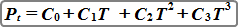Where:
Pt = fraction of 24-hour precipitation
T = elapsed time (hrs)
C0 = coefficient
C1 = coefficient
C2 = coefficient
C3 = coefficient

The list of coefficients for each distribution may be obtained from the NRCS.

Synthetic (Nested) Storms

Many practicing civil engineers use the NRCS 24-hour storms but keep in mind that any storm of any duration can be used with the unit hydrograph method. The Bulletin 71 Huff and the built-in synthetic, nested distributions are good examples and have gained popularity over the years.

The Synthetic Storm option can actually produce an infinite number of design storm hyetographs but for practical reasons, Hydrology Studio limits them to 1, 2, 3, 6, 12 and 24-hour durations. The program uses the rainfall IDF curves to compute depth increments over the time intervals. From this, the design storm is constructed by placing the maximum depth increment near the center of the storm and arranging the other increments in a symmetrical alternating form.

This is the same method used by the SCS years ago to construct their 24-hour storms.

There’s much debate about the usefulness of a 24-hour storm applied to a small urban-like site with Tc’s as low as 15 minutes. The Synthetic Storm offers a good solution in that it can be matched to the site, that is, its total duration can be specified so that it better fits the computed Tc. For example, if Tc is 20 minutes, you could specify a one-hour storm rather than deal with a 24-hr storm. The one-hour storm lasts long enough so that the entire drainage area contributes to flow to the most downstream point. Going beyond Tc only adds unnecessary volume and calculation resources.

Converting the Design Storm to Excess Hyetographs

Regardless of which Design Storm Distribution you are using, including the NRCS, Synthetic, Huff and the Custom storms directly input, the precipitation increments must be converted to excess precipitation. This is where the Curve Number comes in and determines how much of the actual rain is converted into runoff or excess. The following equation is used: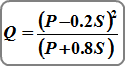Where:
Q = excess volume of precipitation (in)
P = accumulated precipitation (in)
S = potential maximum retention
= (1000 / CN) – 10
CN = SCS curve number

The computed volumes are then converted to excess increments used for the final excess precipitation hyetograph. It is this hyetograph that is used to form the final runoff hydrograph.

Computing the Final Hydrograph

Hydrology Studio computes NRCS Method runoff hydrographs by convoluting a rainfall hyetograph through a unit hydrograph. This method is known as linear super positioning, and means that each ordinate of the rainfall hyetograph is multiplied by each ordinate of the unit hydrograph, thus creating a series of smaller hydrographs. These hydrographs are then summed to form the final runoff hydrograph. See illustration above.